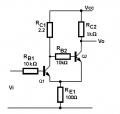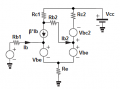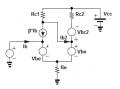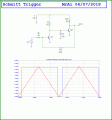# Schmitt trigger threshold voltage

#### Jony130

Joined Feb 17, 2009
5,241
Hi.

I want to ask a question about BJT's version of a Schmitt's trigger.How would you define this two threshold points VL and Vh.

Vh is when Vin rise towards Vcc and Q1 start to conduct Ic1 current and Ic1 pulls current away from the Q2 base. And eventually Q2 start to coming-out from the saturation region and positive feedback kicks-in.

So to be able to find this threshold point I write these equations

How to write the equation for this point?

#### crutschow

Joined Mar 14, 2008
27,414
When the input is low, the current from Q2 is generating a voltage across Re1, creating a turn-on voltage for Q1 equal to that voltage plus Q1's Vbe.
When Q1 turns on, Q2 turns off, reducing the current through Re1, and thus the threshold voltage, producing the Schmitt trigger action.
You just need to write the equations for those two conditions.

Note that the value of Rc1 appears to be much too small for proper circuit operation.

#### MrChips

Joined Oct 2, 2009
23,931
Ask yourself, where is the positive feedback mechanism?

What is different when Vi = VL and Vi = VH?

Edit: Darn! @crutschow gave it away!

#### Jony130

Joined Feb 17, 2009
5,241
When the input is low, the current from Q2 is generating a voltage across Re1, creating a turn-on voltage for Q1 equal to that voltage plus Q1's Vbe. When Q1 turns on, Q2 turns off, reducing the current through Re1, and thus the threshold voltage
But when Q1 turns on Q2 won't cut-off immediately.

The "easiest" part is the situation when the input voltage chnages from Vcc towards the lower threshold voltage VL.
Q1 is saturated and Q2 is cut-off. A Q2 tuns on whne Q1 Vce1 > 0.6V

Heance if I Assume that Vce1 = 0.6V is a Q2 turns on voltage I can easily solve for the VL voltage.

Vcc = Ib1*β*Rc1 + Vce1 + Ib1(β+1)Re

And VL = Ib1*RB1 +Vbe1+ Ib1*(β+1)*Re = ((Vcc - Vce1)* (Rb1 + (β+1)*Re))/(β*Rc1 + (β +1)*Re) + Vbe1

where Vce1 is a Q2 turns-on voltage.

But how big the turn-on voltage should be to start the positive feedback mechanism?

Note that the value of Rc1 appears to be much too small for proper circuit operation.
A typo. It should be 2.2kΩ

Last edited:

#### MrAl

Joined Jun 17, 2014
8,363
Hello Jony,

We could look at this at different levels of detail. The most detailed would be using spice models for the two transistors, but to avoid that a simple approach is to just realize what it takes to get the feedback latching mechanism to start.
The answer to that is when the voltage of Vre1 falls faster than the input voltage. That's because when it falls faster it necessarily makes the input "look higher" and that means it will latch up because it looks like the drive is getting higher and higher all the time even though it may not be changing at all.

An approximation for this would be to simply model each transistor as a diode and a current controlled current source. The CCCS is obviously for the transistor gain, and the diode is to show some of the effect that we see during the near-linear part of the action just before latchup. That should be sufficient to at least show the mechanism although for accuracy we'd need a better model.

For a good working circuit, the near linear region should be narrow.

Last edited:

#### Jony130

Joined Feb 17, 2009
5,241
The CCCS is obviously for the transistor gain, and the diode is to show some of the effect that we see during the near-linear part of the action just before latchup.
For the Vh case (Vh is when Vin rise towards Vcc and Q1 start and Q2 is in saturation) I drawn this equivalent circuit:How you would sovle it for VH ? Or maybe the equivalent circuit is bad?

#### MrAl

Joined Jun 17, 2014
8,363
For the Vh case (Vh is when Vin rise towards Vcc and Q1 start and Q2 is in saturation) I drawn this equivalent circuit:

View attachment 153702

How you would sovle it for VH ? Or maybe the equivalent circuit is bad?
Hi again,

The circuit looks good for the initial topology, and using Vbc2 is interesting because that allows us to find when the second transistor comes out of sat, and that is the point where we have to switch topologies.
I think i might use Vce2 instead though, and just solve for Vbc2. Vce2 would then be set to maybe 0.2v or something like that which would be Vsat for that transistor.
As the input voltage rises, the current ib1 increases and of course that means the base current Ib2 decreases, and eventually Q2 starts to come out of saturation. At that point we would need to change Vce2 to ic2 so that we can get the change in current as the base current decreases. The current ic2 will get lower and thus the voltage across Re will decrease making Vin look higher and thus we see the positive feedback.

So one of the key points is to follow the change in ib2 until Q2 comes out of sat, then switch Vce2 to ic2 and solve for the gain required to produce the saturation voltage of 0.2v (or whatever). At that point the current ib2 will start to follow the input voltage Vin. As Vin increases, ib2 will decrease, etc., and since ic2 decreases vRe gets lower and thus ib1 increases even more and we see the positive feedback.

We might be able to see the action working using the Vbe sources as pictured, but we may have to change them to actual diodes with their non linear curve in order to see everything work right because without that the circuit might be totally linear.

Can you try it like that?
The main thing is to solve for the point where Q2 comes out of sat, then substitute a current source for Q2 as usual, then solve for the current that forces the same Vsat as just before we switched topology. After that Vin can be raised more to see if the circuit snaps into the next saturation mode (opposite to what we started with).
If it continues to be linear, we probably have to use diodes in place of the Vbe sources.

Of course the values used for the components come into play also. It may be better to start with a circuit that is known to work right first and try that first, then move on to whatever you want to accomplish.
With known values, we dont have to predetermine what ratios some of the resistors have to be to get a good ST out of this. For example, RC2 probably has to be lower than RC1, maybe a lot lower, in order to allow Q2 to control the voltage across Re more than Q1 can. Also, then we dont have to solve for a gain we just have to know the value of Rb2 or solve for that instead with fixed gain like 50 for Q2.

If you just want the equations for all this i think i can work them out if you dont want to do that. I am guessing this isnt really homework just something you want to do.

Attached is a pic of the plot with the transistor circuit and with the circuit with the two current sources and two base emitter diodes.
The green output (current source circuit) is similar to the blue output (actual transistor circuit). That at least shows that the method above would be a starting point for an analysis.

Last edited:

#### Jony130

Joined Feb 17, 2009
5,241
To simplify the case a bit I assumed Rb2=0Ωso, the voltage at Q1 colector is equal to the voltage at the Q1 base.

And the equations look like this:

(β + 1) ( (Vcc - Vh)/Rc1 - β*Ib1 ) = (β + 1)/β * (Vcc - Vh + Vbc2)/Rc2

(β + 1)/β *(Vcc - Vh + Vbc2)/Rc2 + (β + 1)* Ib1 = (Vh - Vbe1)/RE

where Vh is a voltage at the Q1 base.

And the solution gives quite good approximation (calculations vs LTspice)For Vbe = 0.7; Vbc2 = 0.55V; β = 100

Vh = 2.18674V ; Ib1 = 0.0435694mA hence

VH = Vh + Ib1*Rb1 = 2.18674V + 0.0435694mA*10kΩ = 2.62V (about 0.1V off from the simulation).

What do you think ?

And yes, I would like to see you approach/equations.

Last edited:

#### MrAl

Joined Jun 17, 2014
8,363
Hello,

I dont see your circuit though, i just see the transistor circuit.

I did a full linear analysis, and this shows that the solution is linear using linear elements. The equation shown is linear with a constant offset, so it is too linear to work the way we want it too. That means diodes have to be introduced which i did not do yet.

The solution shown includes B1 and B2 the Beta for each transistor respectively.
You can try different values if you like.

Also note the addition of R4.

[LATER]
It dawned on me that since we can make a ST with an op amp, we could probably design one using just transistors if we make the gain high enough and just provide positive feedback. The op amp would be used in a non linear mode however but we can get the transistors to do that too. This would be a different approach than we usually see on the web.

Last edited:

#### Jony130

Joined Feb 17, 2009
5,241
I dont see your circuit though, i just see the transistor circuit.
I was useing this equivalent circuitAnd assumed that Q2 leaves the saturation region when IRc1 - Ic1 = Ic2/β

(Vcc - VH)/Rc1 - Ib1/β = ( (Vcc - (VH + Vbc2)) / Rc2 )/β

(VH - Vbe1)/Re = (β + 1)*Ib1 + (Vcc - VH + Vbc2)/Rc2

It dawned on me that since we can make a ST with an op amp, we could probably design one using just transistors if we make the gain high enough and just provide positive feedback. The op amp would be used in a non linear mode however but we can get the transistors to do that too.
But this transistor based Schmitt trigger work on a different principal. In BJT version it works because we have Ic1<Ic2 which correspond to the different Ve voltage.

#### MrAl

Joined Jun 17, 2014
8,363
Hello again,

Ok, i guess you are using Vin=VH then.
That wasnt clear right off.

I might play around with a version that looks more like an op amp circuit just to see what it can do. I think it will be easy to set up too.

#### Jony130

Joined Feb 17, 2009
5,241
Yep. I later on include Rb1 resistor in the calculation

Vth_high = VH+Ib1*Rb1

#### MrAl

Joined Jun 17, 2014
8,363
Yep. I later on include Rb1 resistor in the calculation

Vth_high = VH+Ib1*Rb1
Hi,

Ok thanks.

Here is the circuit i was thinking of, without trying too hard to get any optimum resistor values.
It remains in the linear mode for a very short time so it looks good.
Very simple to understand how it works too, it's just a high gain non linear amplifier with positive feedback ... just like we might do with an op amp or comparator chip.
The output is nearly rail to rail also.
We might add a bias resistor for the base of Q1 for more adjustment range.#### Jony130

Joined Feb 17, 2009
5,241
Yes, this version is a very similar to opamp version. But finding two threshold point won't be easy also

Last edited:

#### MrAl

Joined Jun 17, 2014
8,363
Yes, this version is a very similar to opamp version. But finding two threshold point won't be easy also
Hi again,

I was working on that a little with the 'linear' approximation. I was going to replace the output that supplies the 20k resistor with a voltage source set to the 'previous' state, then solve for the next state or more exact solve for the point where the output starts to change and then go from there.
A problem with the linear version is the output is not clamped, and to see the non linear action it has to be clamped but that is a non linear action. So i may just solve for the 'previous' state then solve for the time it starts to change to the next state, etc.
Interesting though.
I also found that R3 can be eliminated to make it a tiny bit simpler.

#### MrAl

Joined Jun 17, 2014
8,363
Hello again,

Here is the simpler circuit.
The threshold points are not that hard to calculate, approximately. More exact for example the upper threshold input voltage is:
E1=(B1*B2*E3*R2*R3-B2*E3*R1*R3+B2*E2*R1*R3+E3*R1*R2-E2*R1*R2)/(B1*B2*R2*R3)
where
E1 is input voltage,
E2 is supply voltage,
E3 is base emitter voltage assumed, the same for both transistors,
B1 is Beta for Q1,
B2 is Beta for Q2,
other values on the schematic.

However, looking at the circuit we can see the approximate upper threshold is just the drop on the input voltage caused by R1 and R4 and the base emitter diode voltage because that is when Q1 starts to conduct. Add maybe 10 percent and we are even closer:
20/(20+2)*Vin=0.7
Vin=0.77
and adding 10 percent so the transistor conducts more heavily and we get Vin=0.847 .
The more exact value is 0.865 with E2=5, E3=0.7, B1=B2=50.

The lower threshold could be approximated in a similar manner knowing the assumed base emitter voltage.

Note all of the above calculations were using the circuit with current sources and fixed Vbe voltage drops, so it is the linear circuit where the only non linearity is caused by the clamping action of the output (because the limits on the output are 0v and +5v).

Last edited: